## 东风风神 东风A9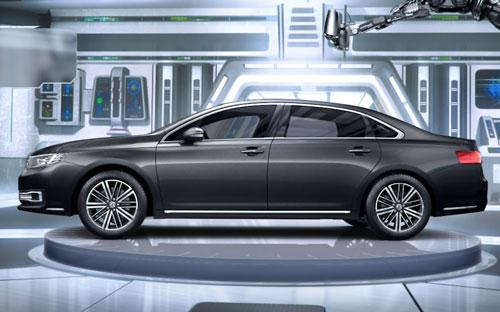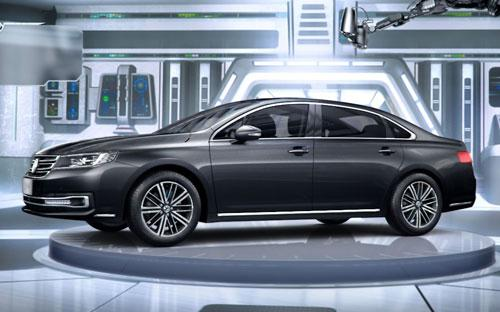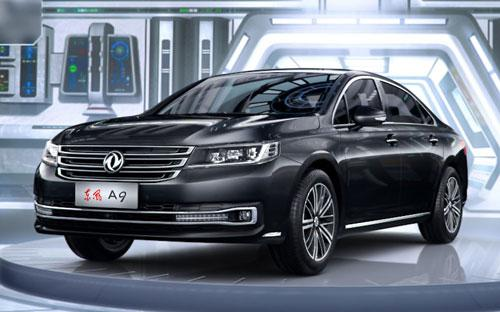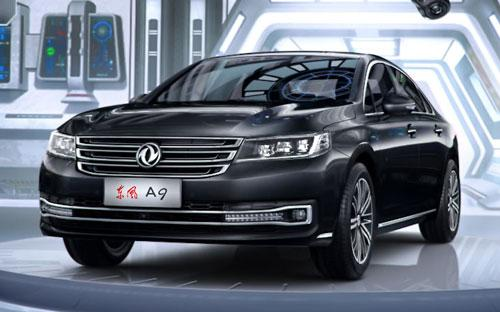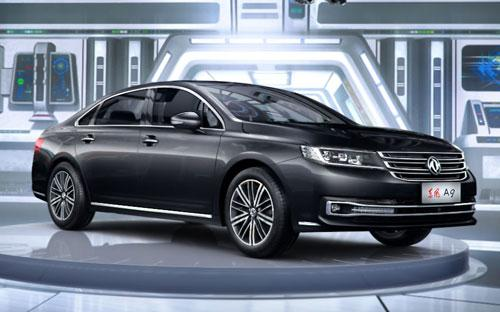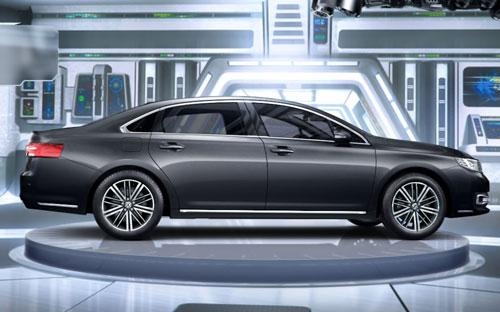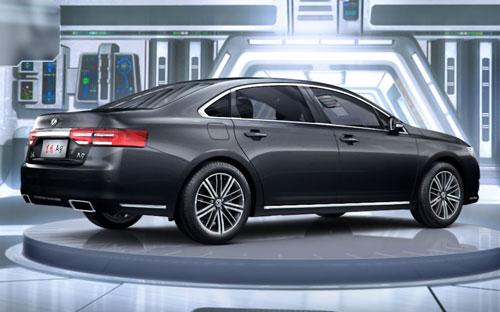3 种颜色可选2016款最低售价：17.97 万元起

5066(mm)1858(mm)1470(mm)##### 配置亮点：
• 胎压监测装置

• ISOFIX儿童座椅接口

• 车身稳定控制(ESC/ESP/DSC等)

• 电动天窗

• 定速巡航

• 后倒车雷达

• 真皮座椅

• GPS导航系统

• 氙气大灯

• 后视镜加热

2016款 1.8T 豪华型 (120张)
• 2016款 1.8T 豪华型 (120张)
• 2016款 1.8T 尊贵型 (151张)
• 2016款 1.8T 旗舰型 (103张)东风风神 东风A9 在售车型

排量 车型 厂商指导价 本地最低报价 购车工具
1.8T 1.8T 豪华型 6挡手自一体 17.97万 17.97万

询底价+对比
1.8T 尊贵型 6挡手自一体 19.97万 19.97万

询底价+对比
1.8T 旗舰型 6挡手自一体 21.97万 21.97万

询底价+对比

东风风神 东风A9 经销商

查看更多 >>
更多 >>

本地降价排名

### 东风风神 东风A9 动力加速

东风A9 0-100公里加速时间分布在 8.5-8.5秒 属于 运动级

动力级别 加速时间 车型
运动级(1款) 8.5s 1.8T 豪华型1.8T 尊贵型1.8T 旗舰型

东风风神 东风A9 视频

东风风神 东风A9 新闻资讯

# 拿奖到手软 东风风神广州车展斩获多项殊荣

行业动态 超过9656次关注

广州车展上，东风风神一举将2016年度车型大奖、年度时尚自主品牌SUV、年度最值得期待自主品牌SUV、年度最受关注紧凑型SUV、中国品牌崛起奖、年度最佳进取品牌等多项...

# 东风A9正式上市 售17.97-21.97万元

上市新车 超过9213次关注

4月12日，东风乘用车官方宣布，旗下全新中大型轿车A9正式上市。东风A9是东风乘用车体系的旗舰轿车，搭载1.8T发动机，共有3个配置车型可选，售价区间为17.97-21.97万元。

# 盘点四月份即将上市的新车 总有一款适合您

即将上市 超过8584次关注

一转眼就到了4月份的第二周，本周将上市7款新车，自主、合资、进口均有，包括比亚迪元、国产XT5、全新思域、风神A9、AX7、蔚揽等。

# 东风风神一季度产销破三万

行业动态 超过9234次关注

阳春三月，生机盎然。近日，记者从东风乘用车公司销售部门获悉，东风风神一季度开票达39087台，同比增长22.8%，其中轿车15655台，同比逆势增长24.0%，SUV23432台，...

# 预计17-22万元 东风A9将于4月10日上市

国产新车 超过8770次关注

2月28日，东风乘用车对外展示了旗舰车型——东风A9，该车将会在今年4月10日正式上市，预售价为17-22万元。

# 定名为9 东风A9底气何在

行业动态 超过8067次关注

对中国自主品牌而言，从“价格竞争”到“品质竞争”，再到“品牌竞争”，这是一条必经之路。从这层意义而言，东风A9不仅是东风品牌的一大跨越，也是一家“国字头”...

猜你喜欢

﻿
• 快速找车
• 选择品牌
• 选择品牌
• A  奥迪
• A  阿斯顿·马丁
• A  阿尔法·罗密欧
• B  宝沃
• B  布加迪
• B  巴博斯
• B  保时捷
• B  宾利
• B  奔驰
• B  宝马
• B  本田
• B  别克
• B  标致
• B  比亚迪
• B  宝骏
• B  北汽制造
• B  北汽新能源
• B  北汽幻速
• B  北汽威旺
• B  北京汽车
• B  奔腾
• B  北汽绅宝
• C  长安
• C  长安商用
• C  长城
• C  昌河
• D  大众
• D  道奇
• D  DS
• D  东南
• D  东风风神
• D  东风风行
• D  东风小康
• D  东风风度
• D  东风
• F  福特
• F  丰田
• F  菲亚特
• F  法拉利
• F  福田
• F  福迪
• F  福汽启腾
• G  观致
• G  广汽传祺
• G  广汽吉奥
• G  GMC
• H  红旗
• H  汉腾汽车
• H  哈弗
• H  哈飞
• H  海格
• H  海马
• H  华颂
• H  黄海
• H  华泰
• H  恒天
• J  吉利汽车
• J  捷豹
• J  Jeep
• J  江淮
• J  江铃
• J  金杯
• J  九龙
• J  金旅
• K  凯翼
• K  凯迪拉克
• K  克莱斯勒
• K  科尼塞克
• K  卡威
• K  开瑞
• L  路虎
• L  林肯
• L  劳斯莱斯
• L  兰博基尼
• L  雷克萨斯
• L  铃木
• L  雷诺
• L  理念
• L  力帆
• L  莲花汽车
• L  猎豹
• L  路特斯
• L  陆风
• M  马自达
• M  MG
• M  MINI
• M  玛莎拉蒂
• M  摩根
• M  迈凯轮
• N  纳智捷
• O  欧宝
• O  讴歌
• O  欧朗
• Q  奇瑞
• Q  起亚
• Q  启辰
• R  日产
• R  荣威
• R  瑞麒
• S  三菱
• S  斯威汽车
• S  萨博
• S  smart
• S  斯柯达
• S  斯巴鲁
• S  思铭
• S  双龙
• S  上汽大通
• S  双环
• T  特斯拉
• T  腾势
• W  沃尔沃
• W  五菱汽车
• W  五十铃
• W  威兹曼
• W  威麟
• X  现代
• X  雪佛兰
• X  雪铁龙
• X  西雅特
• Y  一汽
• Y  英菲尼迪
• Y  英致
• Y  依维柯
• Y  野马汽车
• Y  永源
• Z  众泰
• Z  中华
• Z  中兴
• Z  知豆
• 选择车系
• 选择车系
• 车型对比
• 选择品牌
• 选择品牌
• A  奥迪
• A  阿斯顿·马丁
• A  阿尔法·罗密欧
• B  宝沃
• B  布加迪
• B  巴博斯
• B  保时捷
• B  宾利
• B  奔驰
• B  宝马
• B  本田
• B  别克
• B  标致
• B  比亚迪
• B  宝骏
• B  北汽制造
• B  北汽新能源
• B  北汽幻速
• B  北汽威旺
• B  北京汽车
• B  奔腾
• B  北汽绅宝
• C  长安
• C  长安商用
• C  长城
• C  昌河
• D  大众
• D  道奇
• D  DS
• D  东南
• D  东风风神
• D  东风风行
• D  东风小康
• D  东风风度
• D  东风
• F  福特
• F  丰田
• F  菲亚特
• F  法拉利
• F  福田
• F  福迪
• F  福汽启腾
• G  观致
• G  广汽传祺
• G  广汽吉奥
• G  GMC
• H  红旗
• H  汉腾汽车
• H  哈弗
• H  哈飞
• H  海格
• H  海马
• H  华颂
• H  黄海
• H  华泰
• H  恒天
• J  吉利汽车
• J  捷豹
• J  Jeep
• J  江淮
• J  江铃
• J  金杯
• J  九龙
• J  金旅
• K  凯翼
• K  凯迪拉克
• K  克莱斯勒
• K  科尼塞克
• K  卡威
• K  开瑞
• L  路虎
• L  林肯
• L  劳斯莱斯
• L  兰博基尼
• L  雷克萨斯
• L  铃木
• L  雷诺
• L  理念
• L  力帆
• L  莲花汽车
• L  猎豹
• L  路特斯
• L  陆风
• M  马自达
• M  MG
• M  MINI
• M  玛莎拉蒂
• M  摩根
• M  迈凯轮
• N  纳智捷
• O  欧宝
• O  讴歌
• O  欧朗
• Q  奇瑞
• Q  起亚
• Q  启辰
• R  日产
• R  荣威
• R  瑞麒
• S  三菱
• S  斯威汽车
• S  萨博
• S  smart
• S  斯柯达
• S  斯巴鲁
• S  思铭
• S  双龙
• S  上汽大通
• S  双环
• T  特斯拉
• T  腾势
• W  沃尔沃
• W  五菱汽车
• W  五十铃
• W  威兹曼
• W  威麟
• X  现代
• X  雪佛兰
• X  雪铁龙
• X  西雅特
• Y  一汽
• Y  英菲尼迪
• Y  英致
• Y  依维柯
• Y  野马汽车
• Y  永源
• Z  众泰
• Z  中华
• Z  中兴
• Z  知豆
• 选择车系
• 选择车系
• 选择车型
• 选择车型
• 意见反馈